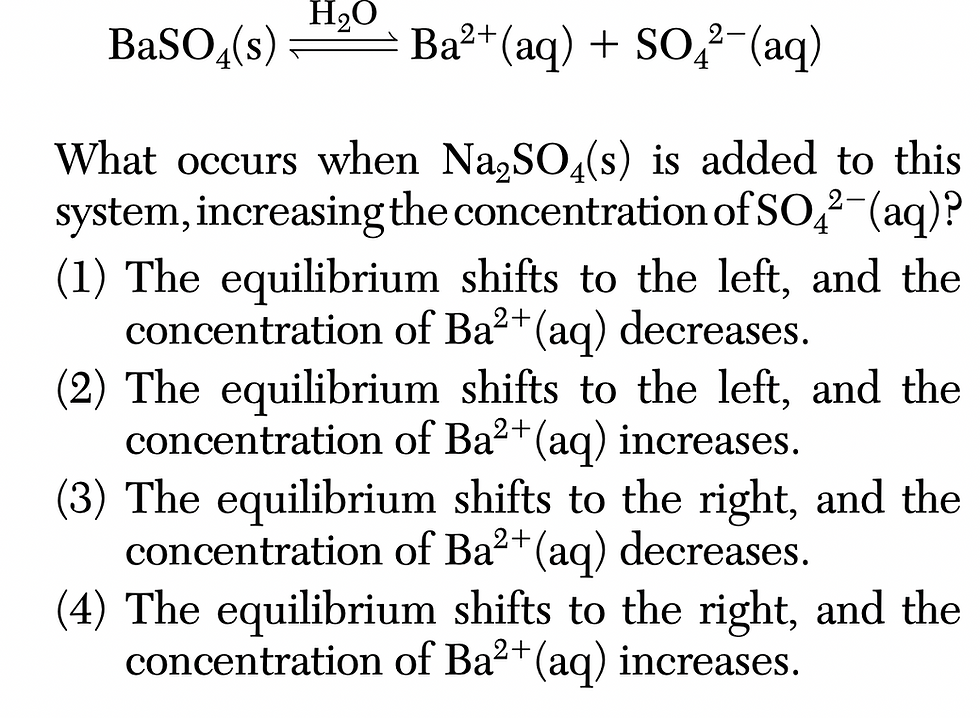top of page
Search

# Given the equation representing a solution equilibrium: What occurs when Na2SO4(s) is added to this

January 2020 NY Regents Chemistry Exam

42 Given the equation representing a solution equilibrium:Solution: Increasing the concentration of SO4^2- shifts the equibrium to the other side in order to decrease it. Equilibrium will shift to the left since SO4^2- is on the right. Shifting to the left will decrease the concentration of Ba2+ ion

Prepare for the Chemistry Regents Exam: HERE